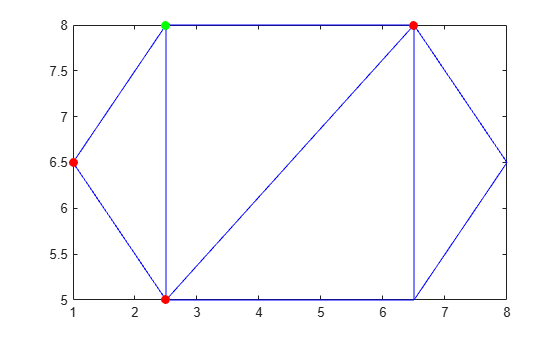# edges

Triangulation edges

## Syntax

``E = edges(TR)``

## Description

example

````E = edges(TR)` returns the triangulation edges as a two-column matrix of vertex identification numbers. Vertex identifications are the row numbers of the triangulation vertices in `TR.Points`. The first column of `E` contains the starting vertex identification of each edge, and the second column contains the ending vertex identification.```

## Examples

collapse all

Load 2-D triangulation data and create a triangulation representation.

```P = [2.5 8.0; 6.5 8.0; 2.5 5.0; 6.5 5.0; 1.0 6.5; 8.0 6.5]; T = [5 3 1; 3 2 1; 3 4 2; 4 6 2]; TR = triangulation(T,P);```

Find the starting and ending vertex identification numbers of the edges in the triangulation.

`E = edges(TR)`
```E = 9×2 1 2 1 3 1 5 2 3 2 4 2 6 3 4 3 5 4 6 ```

Define the coordinates of the first point in the triangulation. Then define the coordinates of the three vertices sharing an edge with the first point (the second, third, and fifth).

```startVert = TR.Points(1,:); endVert = TR.Points([2 3 5],:);```

Plot the triangulation. Plot the first vertex in green, and plot the connecting points along an edge in red.

```triplot(TR) hold on plot(startVert(1,1),startVert(1,2),'g.','MarkerSize',20) plot(endVert(:,1),endVert(:,2),'r.','MarkerSize',20) hold off```## Input Arguments

collapse all

Triangulation representation, specified as a scalar `triangulation` or `delaunayTriangulation` object.

Data Types: `triangulation` | `delaunayTriangulation`